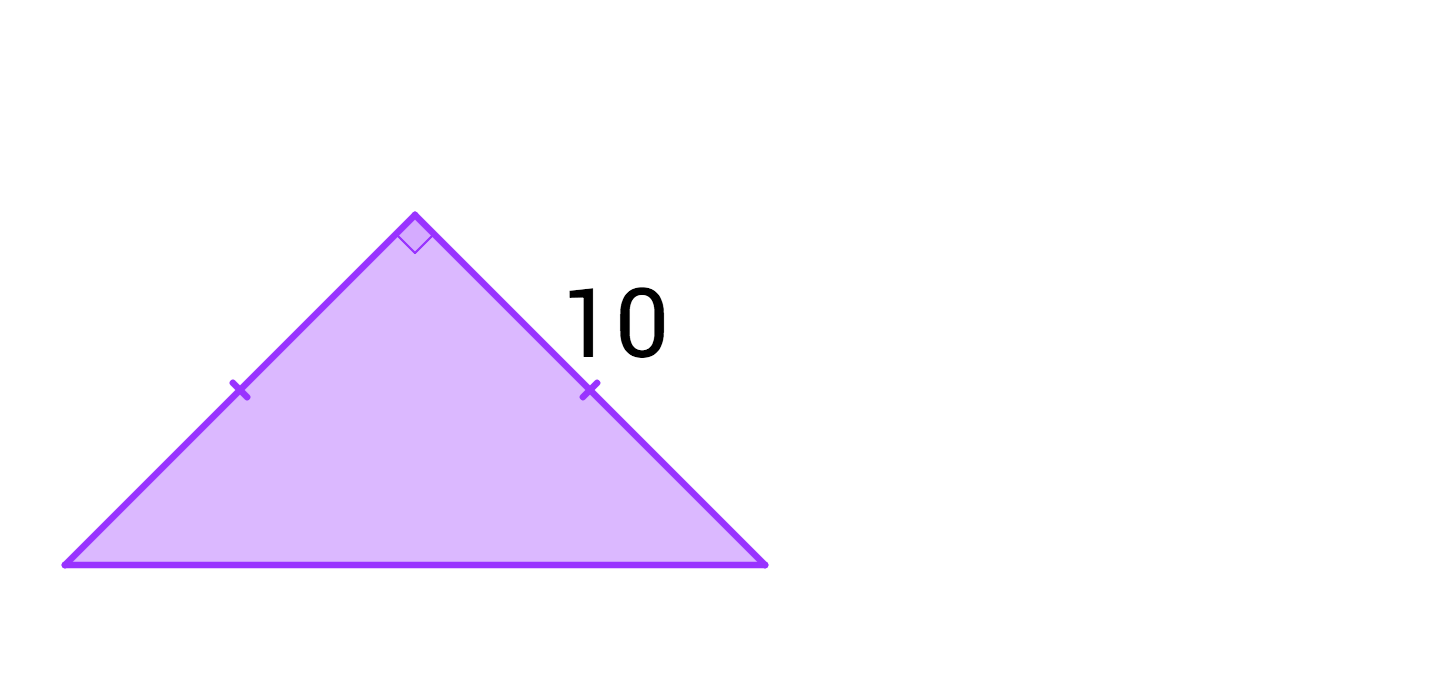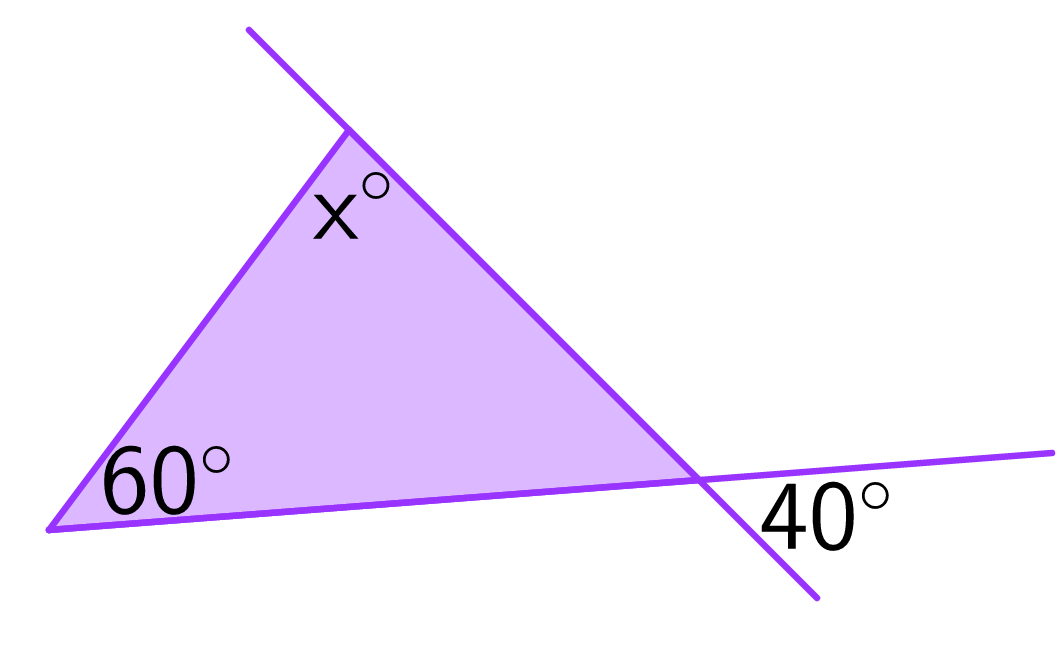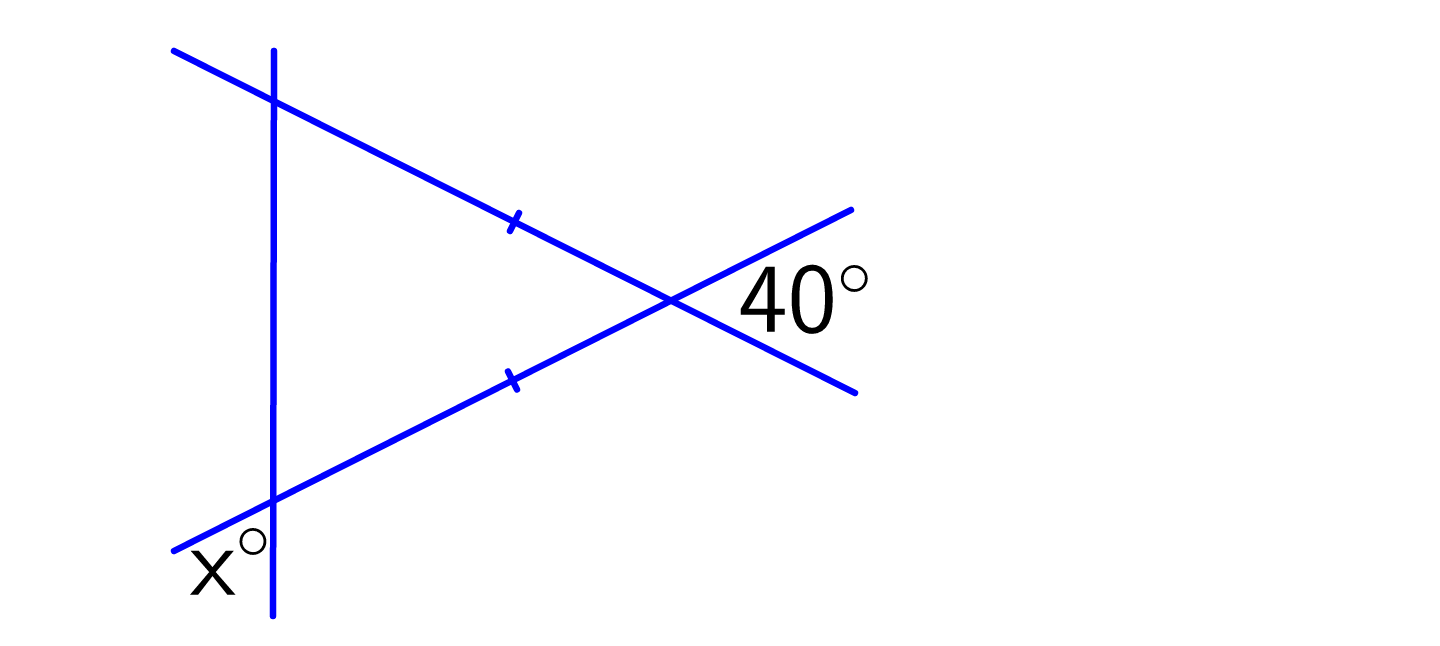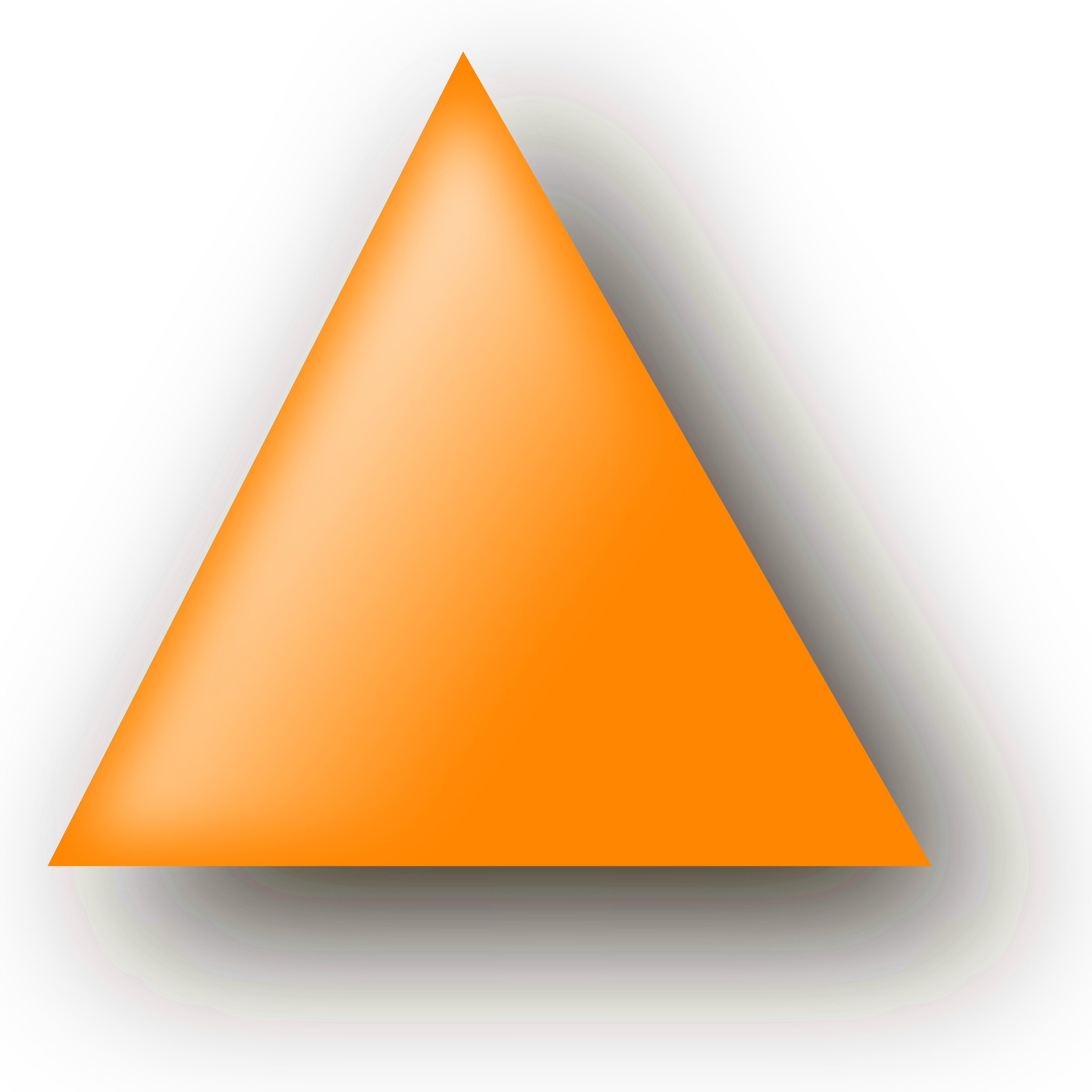Geometry

# Properties of Triangles Lightning QuizWhat is the area of the triangle?What is the value of $$x?$$

Vann's triangle has side lengths of $$a, a + 1, \text{ and } a + 2.$$ Which of these numbers could be the value of $$a?$$What is the value of $$x?$$If a triangle's height is doubled and its base length is halved, the area of the triangle will...

×

Problem Loading...

Note Loading...

Set Loading...